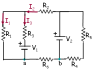# Circuit with 2 Batteries and 6 Resistors

• kjlchem

## Homework Statement

A circuit is constructed with six resistors and two batteries as shown. The battery voltages are V1 = 18 V and V2 = 12 V. The positive terminals are indicated with a + sign, The values for the resistors are: R1 = R5 = 56 Ω, R2 = R6 = 157 Ω R3 = 118 Ω, and R4 = 97 Ω. The positive directions for the currents I1, I2 and I3 are indicated by the directions of the arrows. What is V4?

V=IR

## The Attempt at a Solution

I have been trying to apply Kirchoff's laws for junctions and loops.

I picked 2 loops and got the following equations-

I4R4 +I6R6 = V2

R5I5 + R4I4 + R6I6 + R2I2 + R3I3 = V1

From the junction rule, I got that I1 + I3 = I5 = I2

I'm not sure if this is right though- I5 = I2 = I4 = I6

I don't know what to do next. I think I need another equation.

#### Attachments

•r.png
2.2 KB · Views: 2,999
I think you need to step back from the analysis for a moment and ask yourself, "what is determining the voltage across resistors R4 and R5?"

Your picture is so small it's illegible. Can you post a bigger version?

Your picture is so small it's illegible. Can you post a bigger version?

If you click on it, I think it gets bigger.

Okay, I got that part. Now, to find I3, can I use my equations and Kirchoff's laws?

Never mind! Got it! The problem also asks for the potential difference between V(a) and V(b).

Is this just V=IR where R= R6 and I=I2?

Yes, that's right.

How'd you figure out I3?